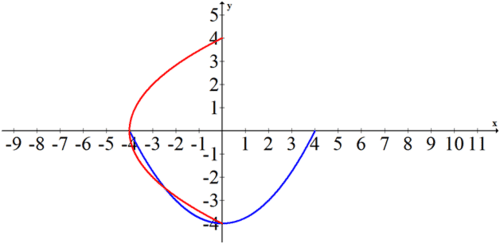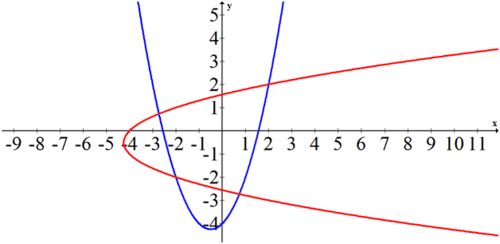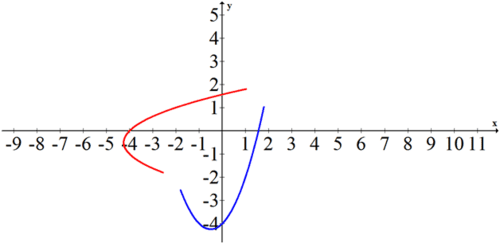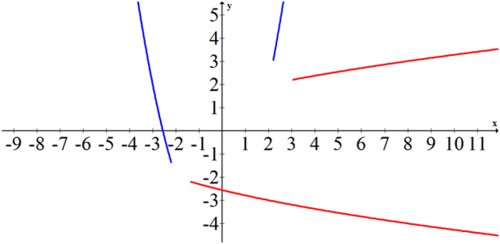# 10.4 Parametric Inverses

$$\newcommand{\vecs}{\overset { \rightharpoonup} {\mathbf{#1}} }$$ $$\newcommand{\vecd}{\overset{-\!-\!\rightharpoonup}{\vphantom{a}\smash {#1}}}$$$$\newcommand{\id}{\mathrm{id}}$$ $$\newcommand{\Span}{\mathrm{span}}$$ $$\newcommand{\kernel}{\mathrm{null}\,}$$ $$\newcommand{\range}{\mathrm{range}\,}$$ $$\newcommand{\RealPart}{\mathrm{Re}}$$ $$\newcommand{\ImaginaryPart}{\mathrm{Im}}$$ $$\newcommand{\Argument}{\mathrm{Arg}}$$ $$\newcommand{\norm}{\| #1 \|}$$ $$\newcommand{\inner}{\langle #1, #2 \rangle}$$ $$\newcommand{\Span}{\mathrm{span}}$$ $$\newcommand{\id}{\mathrm{id}}$$ $$\newcommand{\Span}{\mathrm{span}}$$ $$\newcommand{\kernel}{\mathrm{null}\,}$$ $$\newcommand{\range}{\mathrm{range}\,}$$ $$\newcommand{\RealPart}{\mathrm{Re}}$$ $$\newcommand{\ImaginaryPart}{\mathrm{Im}}$$ $$\newcommand{\Argument}{\mathrm{Arg}}$$ $$\newcommand{\norm}{\| #1 \|}$$ $$\newcommand{\inner}{\langle #1, #2 \rangle}$$ $$\newcommand{\Span}{\mathrm{span}}$$$$\newcommand{\AA}{\unicode[.8,0]{x212B}}$$

You have learned that a graph and its inverse are reflections of each other across the line $$y=x$$. You have also learned that in order to find an inverse algebraically, you can switch the $$x$$ and $$y$$ variables and solve for $$y$$. Parametric equations actually make finding inverses easier because both the $$x$$ and $$y$$ variables are based on a third variable $$t$$. All you need to do to find the inverse of a set of parametric equations and switch the functions for $$x$$ and $$y$$.

Is the inverse of a function always a function?

## Inverses of Parametric Equations

To find the inverse of a parametric equation you must switch the function of $$x$$ with the function of $$y$$. This will switch all the points from $$(x, y)$$ to $$(y, x)$$ and also has the effect of visually reflecting the graph over the line $$y=x$$.

Similar to the inverses regular functions, the inverses of parametric equations are often restricted so that they are also functions. Take the following parametric equations:

$$x=2 t$$

$$y=t^{2}-4$$

To find and graph the inverse of the parametric function on the domain $$-2<t<2,$$ first switch the $$x$$ and $$y$$ functions and graph.

$$x=t^{2}-4$$

$$y=2 t$$

The original function is shown in blue and the inverse is shown in red.## Examples

##### Example 1

Earlier, you were asked if the inverse of a function is always a function. The inverse of a function is not always a function. In order to see whether the inverse of a function will be a function, you must perform the horizontal line test on the original function. If the function passes the horizontal line test then the inverse will be a function. If the function does not pass the horizontal line test then the inverse produces a relation rather than a function.

##### Example 2

Is the point (4,8) in the following function or its inverse?

$$x=2 t^{2}-2$$

$$y=t^{2}-1$$

Try to solve for a matching $$t$$ in the original function.The point does not satisfy the original function. Check to see if it satisfies the inverse.The point does satisfy the inverse of the function.

##### Example 3

Parameterize the following function and then graph the function and its inverse.

$$f(x)=x^{2}+x-4$$

For the original function, the parameterization is:

$$x=t$$

$$y=t^{2}+t-4$$

The inverse is:

$$x=t^{2}+t-4$$

$$y=t$$##### Example 4

An intersection for two sets of parametric equations happens when the points exist at the same $$x, y$$ and $$t$$. Find the points of intersection of the function and its inverse from Example 2.

The parameterized function is:

$$x_{1}=t$$

$$y_{1}=t^{2}+t-4$$

The inverse is:

$$x_{2}=t^{2}+t-4$$

$$y_{2}=t$$

To find where these intersect, set $$x_{1}=x_{2}$$ and $$y_{1}=y_{2}$$ and solve.

\begin{aligned} t &=t^{2}+t-4 \\ t^{2} &=4 \\ t &=\pm 2 \end{aligned}

You still need to actually calculate the points of intersection on the graph. You can tell from the graph in Example $$\mathrm{C}$$ that there seem to be four points of intersection. Since $$t$$ can mean time, the question of intersection is more complicated than simply overlapping. It means that the points are at the same $$x$$ and $$y$$ coordinate at the same time. Note what the graphs look like when $$-1.8<t<1.8$$Note what the graphs look like $$t>2.2$$ or $$t<-2.2$$Notice how when these partial graphs are examined there is no intersection at anything besides $$t=\pm 2$$ and the points (2,2) and (-2,-2) While the paths of the graphs intersect in four places, they intersect at the same time only twice.

##### Example 5

Identify where the following parametric function intersects with its inverse.

$$x=4 t$$

$$y=t^{2}-16$$

$$x_{1}=4 t ; y_{1}=t^{2}-16$$ The inverse is:

$$x_{2}=t^{2}-16$$

$$y_{2}=4 t$$

Solve for $$t$$ when $$x_{1}=x_{2}$$ and $$y_{1}=y_{2}$$.

\begin{aligned} 4 t &=t^{2}-16 \\ 0 &=t^{2}-4 t-16 \\ t &=\frac{4 \pm \sqrt{16-4 \cdot 1 \cdot(-16)}}{2}=\frac{4 \pm 4 \sqrt{5}}{2}=2 \pm 2 \sqrt{5} \end{aligned}

The points that correspond to these two times are:

$$x=4(2+2 \sqrt{5}), y=(2+2 \sqrt{5})^{2}-16$$

$$x=4(2-2 \sqrt{5}), y=(2-2 \sqrt{5})^{2}-16$$

##### Review

Use the function $$x=t-4 ; y=t^{2}+2$$ for #1- #3.

1. Find the inverse of the function.

2. Does the point (-2,6) live on the function or its inverse?

3. Does the point (0,1) live on the function or its inverse?

Use the relation $$x=t^{2} ; y=4-t$$ for $$\# 4-\# 6$$.

4. Find the inverse of the relation.

5. Does the point (4,0) live on the relation or its inverse?

6 . Does the point (0,4) live on the relation or its inverse?

Use the function $$x=2 t+1 ; y=t^{2}-3$$ for $$\# 7-\# 9$$.

7. Find the inverse of the function.

8. Does the point (1,5) live on the function or its inverse?

9. Does the point (9,13) live on the function or its inverse?

Use the function $$x=3 t+14 ; y=t^{2}-2 t$$ for $$\# 10-\# 11$$

10. Find the inverse of the function.

11. Identify where the parametric function intersects with its inverse.

Use the relation $$x=t^{2} ; y=4 t-4$$ for $$\# 12-\# 13 .$$

12. Find the inverse of the relation.

13. Identify where the relation intersects with its inverse.

14. Parameterize $$f(x)=x^{2}+x-6$$ and then graph the function and its inverse.

15. Parameterize $$f(x)=x^{2}+3 x+2$$ and then graph the function and its inverse.

This page titled 10.4 Parametric Inverses is shared under a CK-12 license and was authored, remixed, and/or curated by CK-12 Foundation via source content that was edited to the style and standards of the LibreTexts platform; a detailed edit history is available upon request.